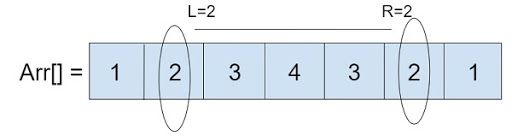# Products of ranges in an array in C

Given with array, L, R, P as an input and the task is to find the ranges between L and R with the product under modulo as output and display it

As given in the figure we have array of elements and L which is a Left value as 2 and R which is the Right value as 2. Now the program must find the products of ranges between them.## Example

Input-:  A[] = { 1, 2, 3, 4, 5, 6 }
P = 29  L = 2 R = 6
Output-: 24
Input-: A[] = {1, 2, 3, 4, 5, 6},
L = 2 R = 5 P = 113
Output-: 7

Approach used in the below program is as follows

• Take the inputs in an array of integer elements, Left value(L), right value(R) and P(prime value)
• Start the traversing of elements from Left value to Right value
• Keep storing the multiplication in a temporary variable
• Keep performing modulo operation with prime value
• Print the final result

## Algorithm

Start
Step 1 -> declare function to calculate product
int calculateProduct(int A[], int L,int R, int P)
declare variable as int i
set L = L – 1
set R = R – 1
declare int ans = 1
Loop For i = L and i <= R and i++
Set ans = ans * A[i]
Set ans = ans % P
End
return ans
Step 2-> In main()
Declare an array as int A[] = { 1, 2, 3, 4, 5, 6 }
Declare variable as int P = 29
Declare variable as int L = 2, R = 6
Print A, L, R, P
Stop

## Example

Live Demo

#include <stdio.h>
int calculateProduct(int A[], int L,int R, int P) {
int i;
//Because array starts with 0 and
//L R starts from 1.
L = L - 1;
R = R - 1;
int ans = 1;
for ( i = L; i <= R; i++) {
ans = ans * A[i];
ans = ans % P;
}
return ans;
}
int main() {
int A[] = { 1, 2, 3, 4, 5, 6 };
int P = 29;
int L = 2, R = 6;
printf("%d", calculateProduct(A, L, R, P));
return 0;
}

## Output

24